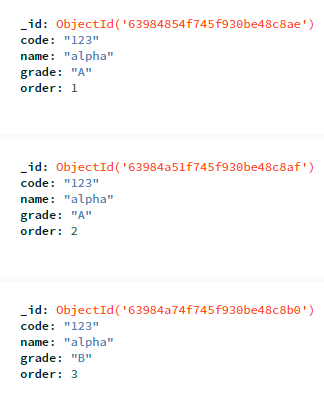# Impossible to use \$switch, \$and and \$match for conditional queries or aggregations

I have got some issues trying to use \$switch and \$match or \$eq for conditionnal cases. Here is my collection of 4 test documents:I would like to query some documents depending on some conditions. But as conditions if/else or switch exist only for aggregation, I use the following aggregation on realm:

``````  doc_switch = collection.aggregate([
{
\$set:{
rm:{
\$switch:{
branches:[
{case:req1,then:1},
{case:req2,then:2},
{case:req3,then:3}
],
default:0
}
}
}
}
])
``````

Where each req is a function of 3 arguments: function(arg_c, arg_n, arg_g) where:

• arg_c: code
• arg_n: name

I want to set the rm attribute based on the switch condition req1, req2 and req3. According to mongodb documentation of switch:

\$switch
Evaluates a series of case expressions. When it finds an expression which evaluates to `true` , `\$switch` executes a specified expression and breaks out of the control flow.

That means, if in my aggregation the case req1 is ok, the \$switch won’t test req2 and so req3.

I have tried different used cases for my conditions as follow:

``````// > error:(InvalidPipelineOperator) Invalid \$set :: caused by :: Unrecognized expression '\$match’
let match1 = {\$match:{"code":arg_c,"name": arg_n , "grade": arg_g}}
let match2 = {\$match:{"code":arg_c,"name": arg_n }}
let match3 = {\$match:{"code":arg_c}}

let and1 = {\$and:[{"code":arg_c},{"name": arg_n},{"grade": arg_g}]}
let and2 = {\$and:[{"code":arg_c},{"name": arg_n}]}
let and3 = {\$and:[{"code":arg_c}]}

// > error:(InvalidPipelineOperator) Invalid \$set :: caused by :: Unrecognized expression '\$match’
let matchand1 = {\$match:{\$and:[{"code":arg_c},{"name": arg_n},{"grade": arg_g}]}}
let matchand2 = {\$match:{\$and:[{"code":arg_c},{"name": arg_n}]}}
let matchand3 = {\$match:{"code":arg_c}}

let andmatch1 = {\$and:[{\$match:{"code":arg_c,"name": arg_n , "grade": arg_g}}]}
let andmatch2 = {\$and:[{\$match:{"code":arg_c,"name": arg_n }}]}
let andmatch3 = {\$and:[{\$match:{"code":arg_c}}]}

let andmatchmatch1 = {\$and:[{\$match:{"code":arg_c}},{\$match:{"name": arg_n}} , {\$match:{"grade": arg_g}}]}
let andmatchmatch2 = {\$and:[{\$match:{"code":arg_c}},{\$match:{"name": arg_n }}]}
let andmatchmatch3 = {\$and:[{\$match:{"code":arg_c}}]}

// Erreur d'expression relevée par Realm
//let eq1 = { {\$eq:["code",arg_c]},{\$eq:["name", arg_n]},{\$eq:["grade", arg_g]}}
//let eq2 = { {\$eq:["code",arg_c]},{\$eq:["name", arg_n]}}
//let eq3 = { {\$eq:["code",arg_c]}}

let andeq1 = { \$and:[{\$eq:["code",arg_c]},{\$eq:["name", arg_n]},{\$eq:["grade", arg_g]}]}
let andeq2 = { \$and:[{\$eq:["code",arg_c]},{\$eq:["name", arg_n]}]}
let andeq3 = { \$and:[{\$eq:["code",arg_c]}]}

// Chosen case
req1 = andmatchmatch1
req2 = andmatchmatch2
req3 = andmatchmatch3

``````

Depending on the request, I have summariezd the resultas as follow in a tab:

I manually get rid of the _id attributes for readibility.
I hope it’s still readable enoughSo basicaly, for all the used cases tested, either there are no match, either the value I have got for the new rm attribute is the wrong and the same for each document.

Is someone know a solution, or know why it doesn’t work.

I hope my issue is clear enough.

Damien

Hello @Damien ,

Welcome to The MongoDB Community Forums!The reason you are getting below error while using \$match in \$switch is that, the `case` field of \$switch is expected to be any valid expression that resolves to a `boolean` . If the result is not a `boolean` , it is coerced to a boolean value. \$match is not an expression (provides `document` as result and not a boolean value) but an aggregation stage.

Invalid \$set :: caused by :: Unrecognized expression ‘\$match’

I would recommend you to go through Expressions in MongoDB to learn more about what are valid expressions and how one can use them to their advantage.

Regards,
Tarun

1 Like

Thank you for your answer @Tarun_Gaur . I didn’t really think about if the \$match was doing a boolean response or something else. I think i will try again to do my aggregation by another way with that in mind.

1 Like

This topic was automatically closed 5 days after the last reply. New replies are no longer allowed.